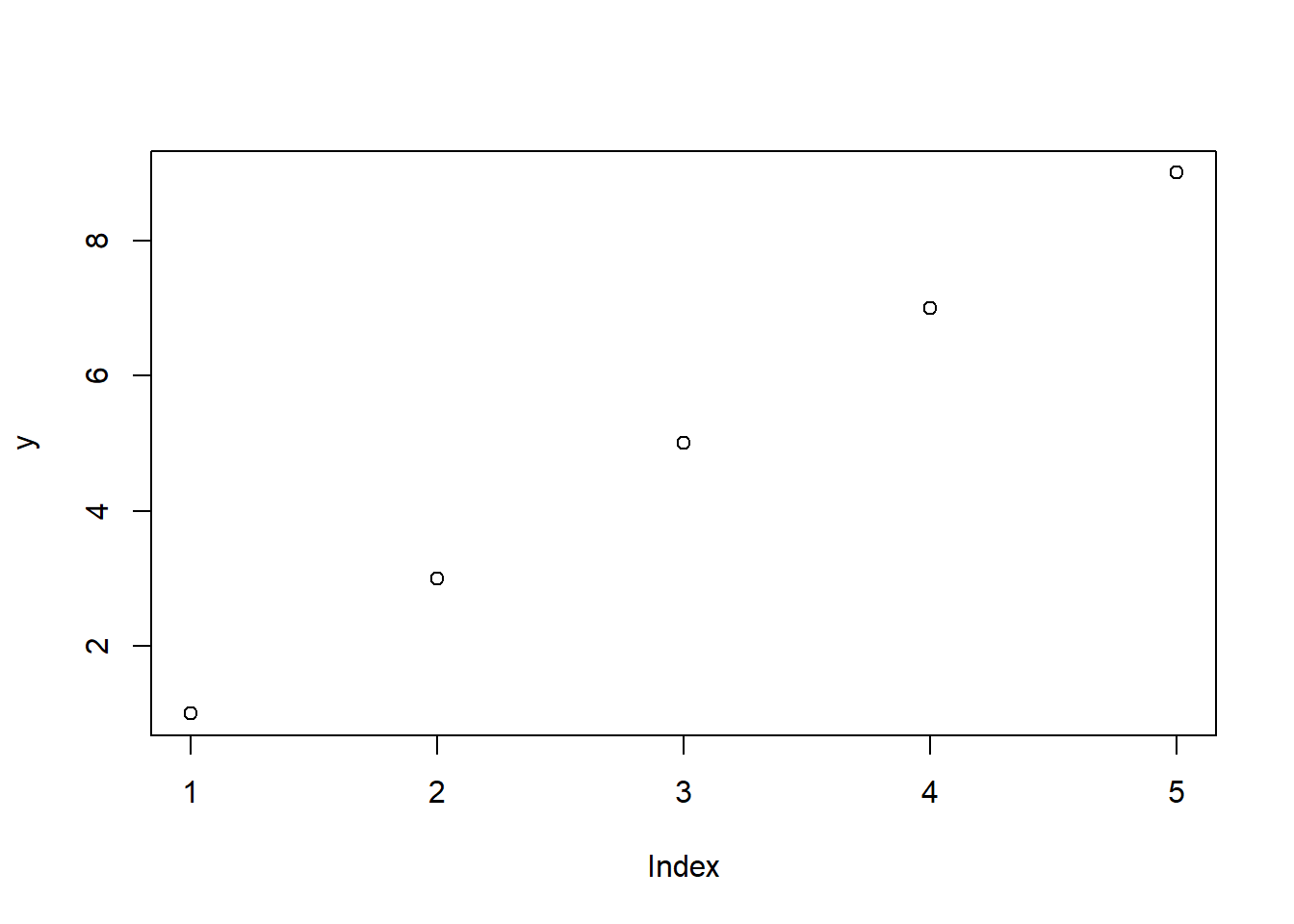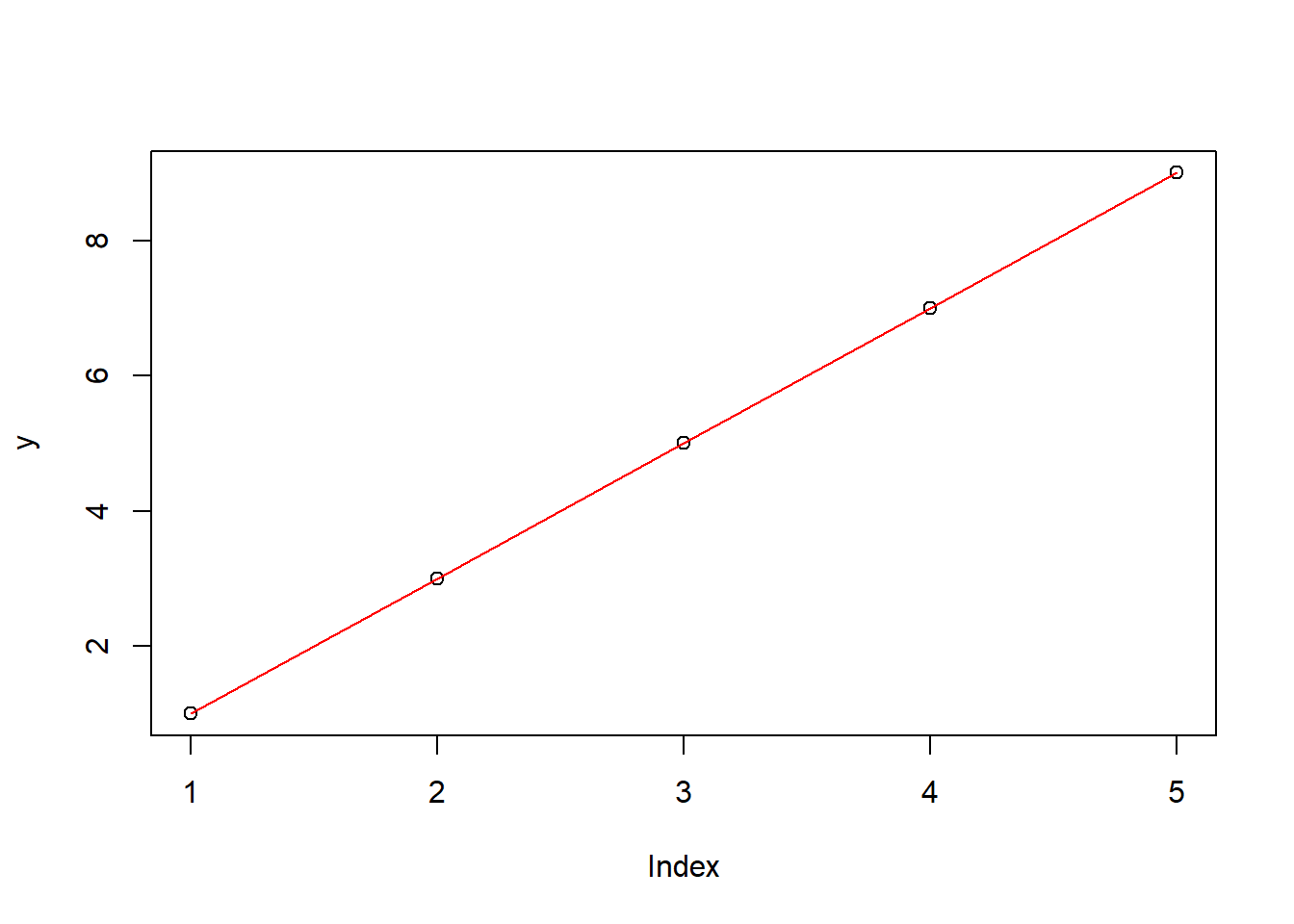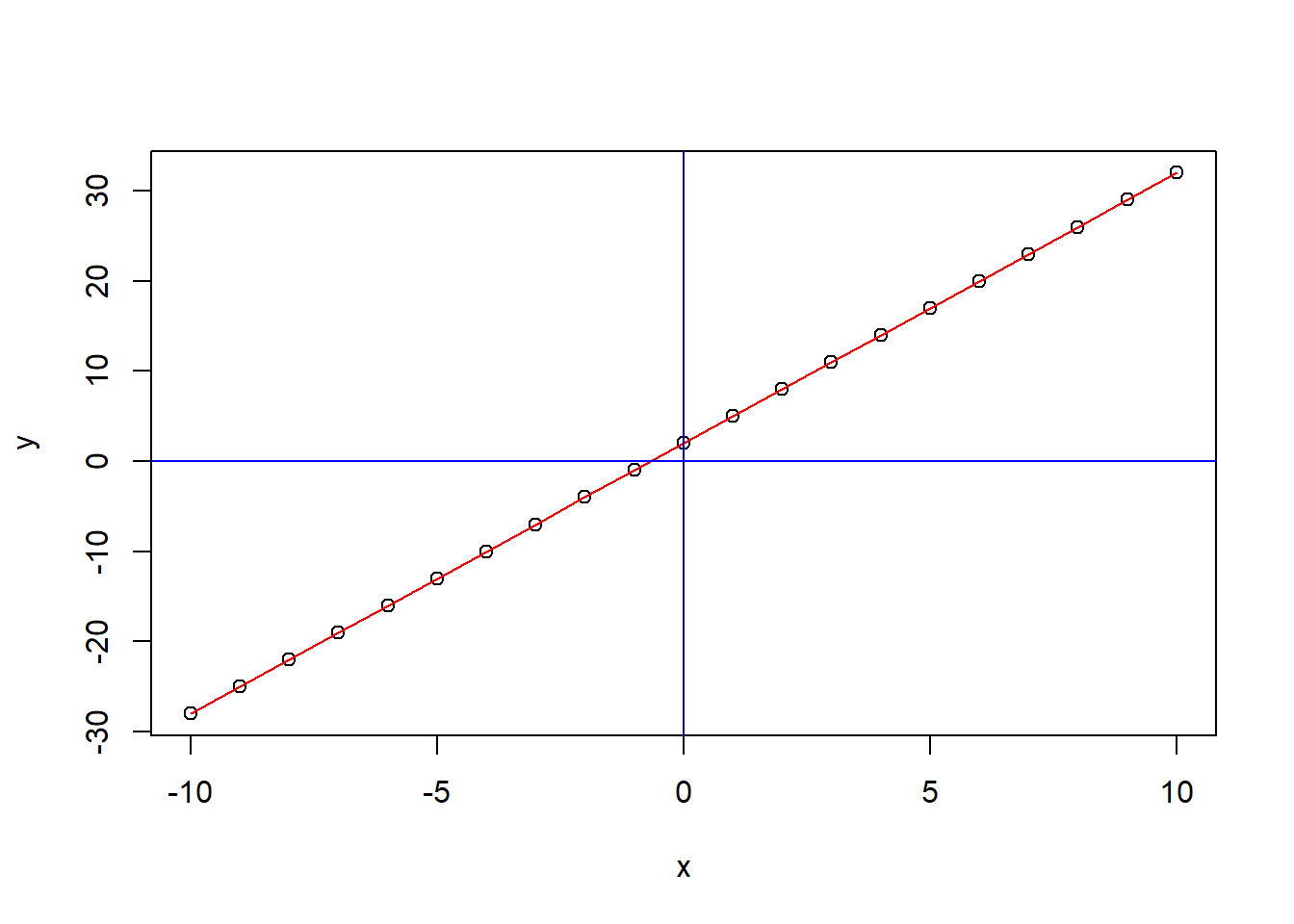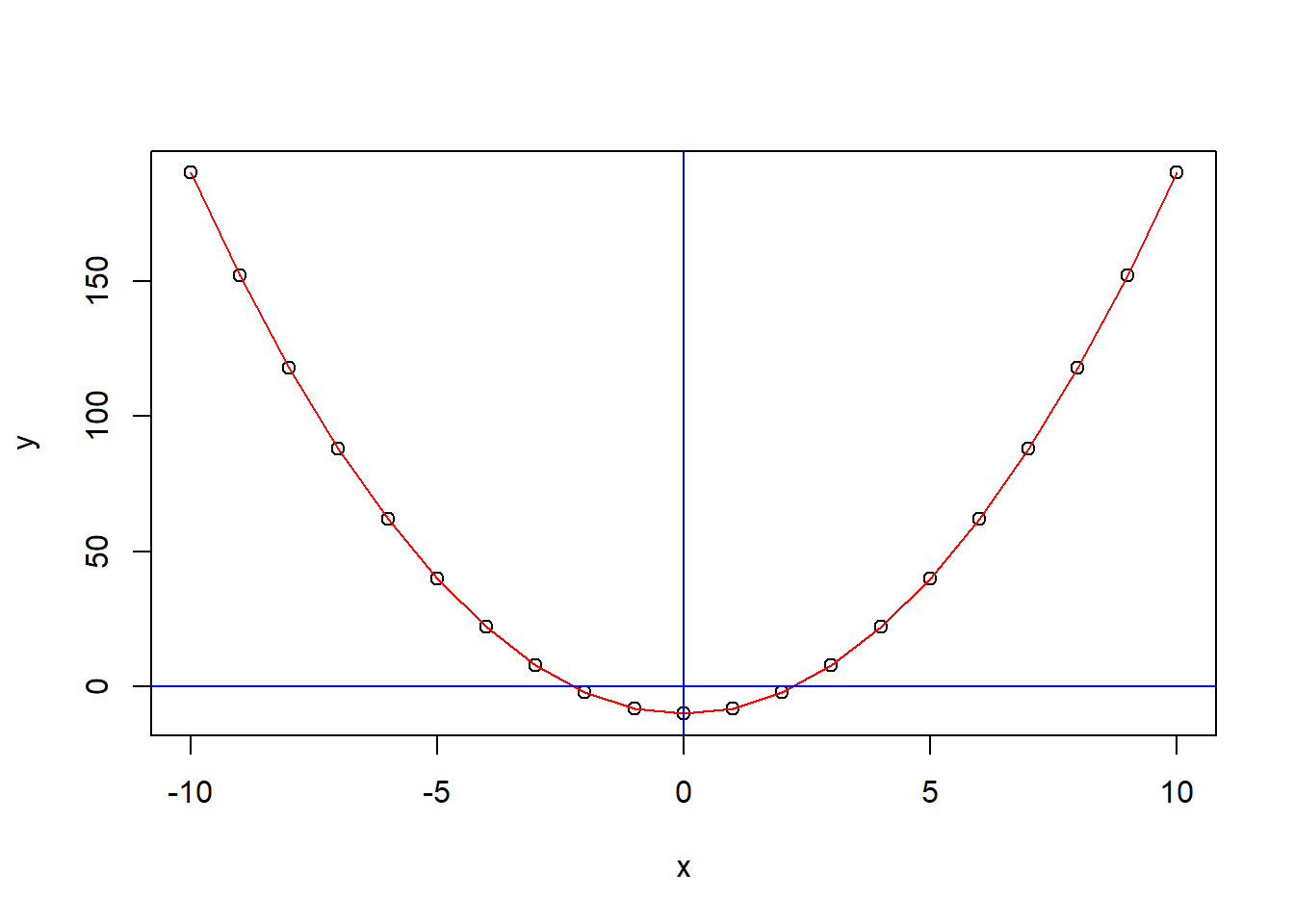# 使用R语言示意初中函数曲线

## 简单的数学计算

### 加减乘除：+ - * /

1+1; 4-2; 3*5; 9/2
##  2
##  2
##  15
##  4.5

### 绝对值abs()、整除%/%、余数%%

abs(5*(-10)); 25%/%3; 25%%3
##  50
##  8
##  1

### n次方(幂) ^n、 开平方 sqrt()、开n次方^(1/n)

2^3 # 2的3次方
##  8
sqrt(9) # 9开平方
##  3
8^(1/3) # 8开3次方
##  2

## 赋值给对象

x = 3 #这是把x这个对象，赋值为3
x
##  3
x = x+1 # x增加了1，变成了4
x
##  4
y = 1:10 # 把y这个对象赋值为1到10，这10个数，一串
y
##    1  2  3  4  5  6  7  8  9 10
y = y+1 #这一串数每一个都加1，变成了2到11.
y
##    2  3  4  5  6  7  8  9 10 11

y = x #则y被赋予之前x的值，即4，成了一个数了
y
##  4

## 在坐标系中表示数值

y = seq(1, 10, 2) # seq函数产生序列，三个参数分别为从哪里开始，到哪里结束，以多大幅度

plot(y)plot(y)
lines(1:5, y,col='red')### 函数

#### 一次函数

yicihanshu = function(x) 3*x + 2 # 这就定义了一个函数，能够代入具体x求值，如代入x=3，结果应该是11.

yicihanshu(3)
##  11

x = seq(-10, 10, 1) # x取从-10到10的整数，即-10、-9、-8……8、9、10.
y = yicihanshu(x) # 分别求得对应的y值

plot(x,y) # 可以看到这些点
lines(x,y, col="red") # 连上线
abline(h = 0, v = 0, col = "blue") # 添加坐标轴位置，便于观察#### 二次函数

x = seq(-10, 10, 1)
ercihanshu = function(x) 2*x^2 -10
y = ercihanshu(x)
plot(x,y)
lines(x,y, col = "red")
abline(h = 0, v = 0, col = "blue")